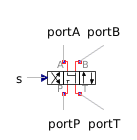PID - MapleSim Help

PIDDescription

The PID component models an ideal proportional-integral-derivative (PID) controller. This controller differs from the ones normally used in the industrial setup as the proportional gain, k, is decoupled from the system. This does not affect the integral and the derivative gain performance. For a more practically useful PID-controller, use the Lim PID component.

The equation of this controller is $y=k\left(1+\frac{1}{{T}_{i}s}+{T}_{d}s\right)u$ where the derivative is estimated numerically based on the time step, ${N}_{d}$. The greater the value of ${N}_{d}$, the better the estimate of the derivative.

The Signal Size parameter allows the block to operate on a vector of signals rather than a single signal.Initialization

The PID block can be initialized in different ways controlled by parameter initType. DoNotUse_InitialIntegratorState is added for backward compatibility reasons.

 initType Integrator initType Derivative initType NoInit NoInit NoInit SteadyState SteadyState SteadyState InitialState InitialState InitialState InitialOutput and initial equation: y = y_start NoInit SteadyState DoNotUse_InitialIntegratorState InitialState NoInit

In many cases, the most useful initial condition is SteadyState because initial transients are no longer present. If $\mathrm{initType}=\mathrm{InitPID}\cdot \mathrm{SteadyState}$, then in some cases difficulties might occur. The reason is the equation of the integrator, $\stackrel{.}{y}=ku$. The steady state equation, $\stackrel{.}{x}=0$ leads to the condition that the input to the integrator is 0. If the input $u$ is already (directly or indirectly) defined by another initial condition, the initialization problem is singular (that is, has none or infinitely many solutions). This situation occurs often in mechanical systems, where, for example, $u=\mathrm{desiredSpeed}-\mathrm{measuredSpeed}$. Because speed is both a state and a derivative, it is natural to initialize it with 0. As sketched, this is not possible. The solution is to not initialize ${u}_{m}$ or the variable that is used to compute ${u}_{m}$ by an algebraic equation. If the parameter Limits At Initial is $\mathrm{false}$, the limits at the output of this component are removed from the initialization problem, which leads to a much simpler equation system. After initialization has been performed, it is checked with an assert whether the output is in the defined limits.Connections

 Name Description Modelica ID $u$ Real input vector u $y$ Real output vector yParameters

 Name Default Units Description Modelica ID Signal Size $1$ Dimension of input and output signals signalSize k $1$ $1$ Gain k ${T}_{i}$ $0.5$ $s$ Time Constant of Integrator Ti ${T}_{d}$ $0.1$ $s$ Time Constant of Derivative block Td ${N}_{d}$ $10$ The greater ${N}_{d}$, the more ideal the derivative component Nd Initial Values NoInit Type of initialization; see Initialization section initType ${x}_{{i}_{0}}$ $0$ Initial or guess value for the output of the integrator component xi_start ${x}_{{d}_{0}}$ $0$ Initial or guess value for state of derivative component xd_start ${y}_{0}$ $0$ Initial value of output y_start